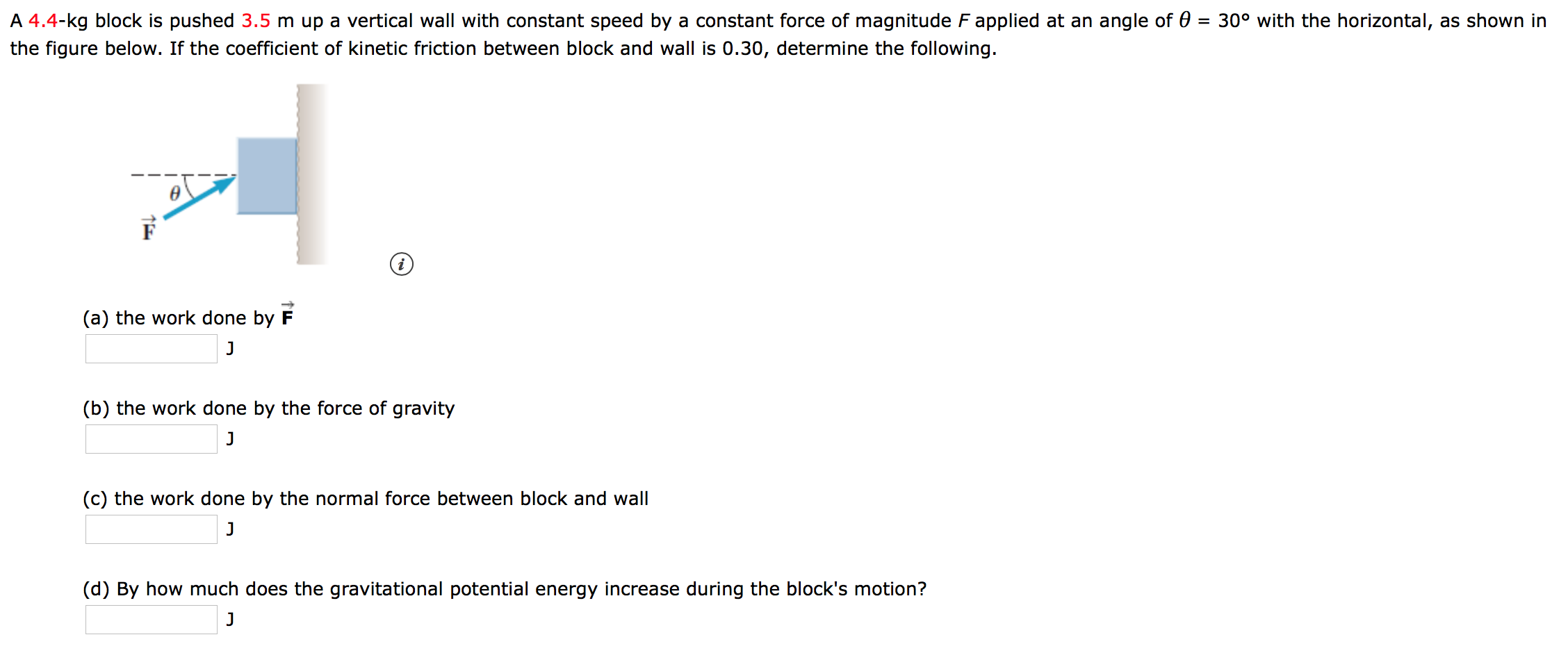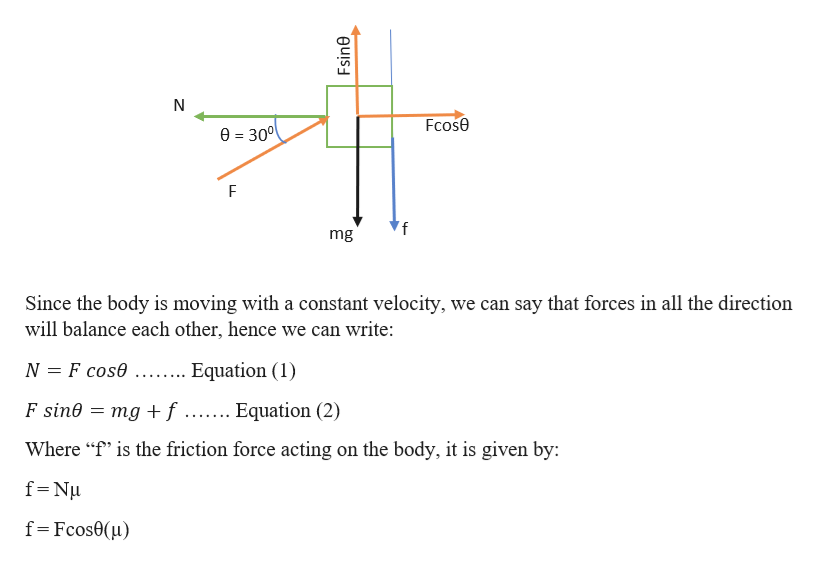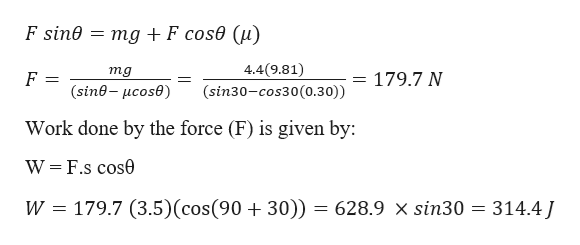# A 4.4-kg block is pushed 3.5 m up a vertical wall with constant speed by a constant force of magnitude F applied at an angle of 0 = 30° with the horizontal, as shown inthe figure below. If the coefficient of kinetic friction between block and wall is 0.30, determine the following.(a) the work done by FJ(b) the work done by the force of gravityJ(c) the work done by the normal force between block and wallJ(d) By how much does the gravitational potential energy increase during the block's motion?J

Questionhelp_outlineImage TranscriptioncloseA 4.4-kg block is pushed 3.5 m up a vertical wall with constant speed by a constant force of magnitude F applied at an angle of 0 = 30° with the horizontal, as shown in the figure below. If the coefficient of kinetic friction between block and wall is 0.30, determine the following. (a) the work done by F J (b) the work done by the force of gravity J (c) the work done by the normal force between block and wall J (d) By how much does the gravitational potential energy increase during the block's motion? J fullscreen
check_circleExpert Solution
Step 1

Given information:

Mass of the block (m) = 4.4 kg

Coefficient of friction between the block and the wall (µ) = 0.30

The distance the block travelled up the wall (s) = 3.5

Angle of the force applied (θ) = 300

Step 2help_outlineImage TranscriptioncloseFcose 0=300 'f mg Since the body is moving with a constant velocity, we can say that forces in all the direction will balance each other, hence we can write: N F cose Equation (1) = mg +f ....... Equation (2) F sine EEE Where "f" is the friction force acting on the body, it is given by: f Nu f Fcos0(u) Fsine fullscreen
Step 3

From equation (2) w...help_outlineImage TranscriptioncloseF sin0 = mg +F cos0 (u) 4.4(9.81) mg = 179.7 N (sine-ucose) (sin30-cos30(0.30)) Work done by the force (F) is given by: W F.s cos0 179.7 (3.5) (cos(90 + 30)) = 628.9 x sin30 314.4J W fullscreen

### Want to see the full answer?

See Solution

#### Want to see this answer and more?

Solutions are written by subject experts who are available 24/7. Questions are typically answered within 1 hour*

See Solution
*Response times may vary by subject and question
Tagged in

### Work,Power and Energy